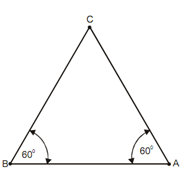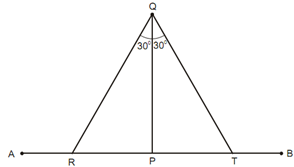## Construct an Equilateral Triangle Assignment Help

Assignment Help: >> Triangle - Construct an Equilateral Triangle

Construct an Equilateral Triangle:

To Construct an Equilateral Triangle when the Length of the Side is given

Employ a T-square and a set-square only. Along with a T-square, draw a line AB of the given length. By using a set-square with angles of 30o - 60o and a T-square, draw a line by A making 60o angle along with AB. Similarly, via B, draw a line making the same angle (60o) along with AB and intersecting the first line at C. ABC is the required triangle.For Constructing an Equilateral Triangle of a Given Altitude

Along with a T-square, draw a line AB of any length. From a point P on AB, draw, along with a set-square, vertical PQ equal to the given altitude. Along with T-square and30o - 60o set square, draw lines via Q on both sides, making 30o angle along with PQ and cutting AB at R and T. QRT is the essential triangle.#### Assured A++ Grade

Get guaranteed satisfaction & time on delivery in every assignment order you paid with us! We ensure premium quality solution document along with free turntin report!

All rights reserved! Copyrights ©2019-2020 ExpertsMind IT Educational Pvt Ltd